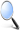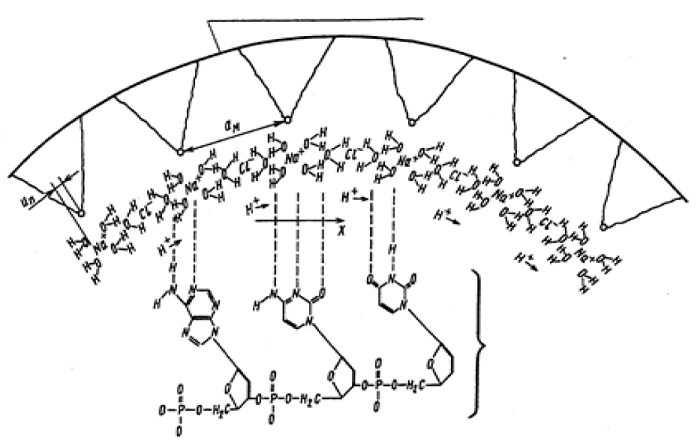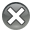Edelweiss Applied Science and Technology is an open access journal, our journal has a wide scope of topics related to science and technology, which are not limited to Basic Science, life science, computer science, environmental science, chemical science, electrical science, electronic engineering, industrial engineering, material science, astronomy and astrophysics.

# Edelweiss Applied Science and Technology (ISSN: 2576-8484)Research Article :

## Electrochemical Approach in Description of a Nerve Impulse

Andrey Berezin, Alexander Shmid

### Abstract

Nerve impulse has been simulated as an ion–acoustic breather containing the internal FPU recurrence spectrum. The strong electrolyte of intracellular fluid is interpreted as dense plasma where electroacoustic solitons can propagate. The dynamics of sodium ion concentration is described by the sine-Gordon equation while the dynamics of proton concentration is done by the Korteweg de Vries equation. The system of these coupled equations has a breather solution having an intrinsic structure with the Fermi–Pasta–Ulam recurrence. Its spectrum possesses a dynamic memory for the initial conditions of its modes. Such breathers can be generated in firing process of a neuron. The information capacity of each breather can reach 1014 bits.

### Introduction

Traditionally, the electrical activity of a single neuron is described within the framework of the Hodgkin-Huxley model , where, in accordance with the Hartley formula, the information capacity of a single spike is bit. In other words, a neuron is considered as a system with two states, and all the complexity of a brains ability to process information is explained by the presence of numerous parallel processing paths. However researches have reported correlations between learning and changes in ribonucleic acid (RNA) and protein in neurons . These data supported a hypothesis  that a single neuron, based on the number of triplets existing in its RNA molecule can sustain an information capacity up to  bits.

The purpose of this paper is to develop a neuronal model based on the complex FPU recurrence spectrum, interpreted as an information carrier. The FPU recurrence phenomenon was first reported in 1955  as a result of a computer simulation of the oscillatory dynamics in a chain of non-linearly coupled vibrators with fixed ends. In contrast to logical expectations, this system did not have a tendency toward equipartition of energy among its degrees of freedom. Instead of this, a periodical recurrence of the initial perturbation energy spectrum was observed. Later , it was established that the FPU phenomenon has two types of recurrence: simple and complex. In a simple recurrence, an almost perfect periodic return of energy spectra is observed in the system, whereas in a complex version, the periods not stable and Fourier modes exhibit complex sharing and regrouping of energy.

This interesting behavior of the FPU chain stimulated a number of investigations of the system [6,7,8,9]. The first theoretical description of the problem was done by Zabusky and Kruskal , who showed that FPU recurrence can be described by the Korteweg-de Vries (KdV) equation with periodic initial conditions. Another team of researchers,

Yuen and Lake, were able to describe FPU recurrence within the framework of the Nonlinear Schrodinger Equation (NLS) with periodic boundary conditions . Same authors reported about very interesting property of the FPU recurrence to "remember" the initial conditions for the active modes of its spectrum, reproducing them periodically in the FPU spectrum. In another study of the FPU problem , it was proposed that the exchange of energy between modes of the system is connected with a transition to strong stochastisity. In discussing early investigations of FPU recurrence, it is necessary to emphasize that the original vibrators chain had fixed ends . But with open ends, the chain also can represent a theoretically interesting autonomous distributed system, responsive to initial perturbations. As a first step of our study we tried to consider neural activity using a plasma approach.

### Neural activity model

We shall consider a neuron as a volume containing the strong electrolyte of intracellular fluid in which RNA molecule plays the role of neural information carrier. Additionally we assume that all potassium ions are electrically neutralized by forming ionic couples with hydroxyl groups that provides an overbalance of protons in the electrolyte of the neuronal intracellular fluid. Such assumptions allow us to analyze the proton dynamics by applying the simplified cluster model of the seawater strong electrolyte suggested by Frank and Wen . In this model, every sodium ion is surrounded by ionic atmosphere consisting of four water molecules and every chlorine ion has two water molecules in its atmosphere (Figure 1).Figure 1: Plasma model of a neuron.

In a spherical volume of a neuron, consider one-dimensional chain of Na+ and Cl- ions near the lower layer of neuronal membrane as illustrated in Figure 1. Depolarization of the membrane creates a nonlinear proton concentration wave due to the "croquet" mechanism of proton transport between water molecules, suggested by Bernal . The electric potential created by the combined sodium ion and the chlorine ion and the chlorine ion atmosphere can be defined within the framework of the Debye-Hukkel theory as a sum of repelling and attracting parts :

Since the duration of a nerve impulse constitutes units of milliseconds  we can formally evaluate the single spike information capacity applying Kotelnikovs theorem  as follows:

Here, t = S/cs, where S = L/sinais the soliton length, ais the resonator spirality angle, c= 10m×s–1, S = 10 m, L = 10 cm, and sina = 0.1.

The next step is to show how this high frequency signal can be embedded into the structure of the action potential. For this purpose, we shall consider a neuron membrane as a liquid crystal containing a

lipid bilayer  (Figure 1). Accounting the distance between the lipid molecules in the membrane layer and the distance between sodium ions in the chain (Figure 1) to be about 4.7 A [16,21], we can suggest the following mechanism of interaction between high frequency proton concentration wave and the ionic wave of the action potential.

Consider the sodium chain shifted at a distance U relative to the equilibrium positions of the lipid molecules of the membrane lower layer (Figure 1). Such a picture allows applying the approach developed by Frenkel and Kontorova  for the analysis of dislocations dynamics in crystals.

We define  potential effecting n-th sodium ion from the immovable chain of lipid molecules in the neuron membrane layer (Figure 1) as:

Where - is the distance between neighboring lipid molecules in the membrane layer,  - relative position of the lipid molecule.

In this case the dynamics of the sodium ion shift can be described by the following equation :

Where- is the elastic constant for the relative shift of the sodium ions.

Now using the continuum approximation  and introducing a function proportional to the modulation of the sodium ion density in a form:

We obtain the sine-Gordon equation:

The dimension of a neuron will define the boundary conditions for Eq. (10) by analogy with a long transmission line open on both ends, which was used for simulation of the Josephson junction dynamics :As shown by Fulton  for chains with limited length described by the sine-Gordon equation there exists an energy exchange between soliton and plasma solution. This type of Eq. (10) solution represents in the limit the standing waves which are close to the knoidal waves of the KdV . These properties of Eq. (10) give an opportunity to apply the experimentally established plasma phenomenon of locking high frequency electric field by the low density plasma regions . Within the framework of this approach we can interpret a spike as a locker of high frequency proton concentration waves within the body of the sodium ion concentration waves, because the strong electrolyte of the neuron intracellular fluid can be considered as a dense plasma. So the nerve spike forming process can be described by the system of coupled sine-Gordon and KdV equations:

where,  < 1 - are the coefficients of interaction between sodium ion concentration waves and proton ones.

In accordance with the results of Ikezi paper , in which a similar pair of equations was studied, the solution of the system (13) represents a soliton with internal oscillatory structure. Parametrical coupling of equations in (13) provides an effective energy exchange . We looked for the systems (13) solution having the following considerations. Since periodical initial conditions imposed on the KdV equation resulted in the model of Zabusky and Kruskal in forming the FPU solution we considered the influence of intermittent KdV solitons on the SG equation as a perturbing factor. Soliton of the SG equation captures the modes of this external influence. The number of captured modes is connected with the asymptotic phase shift . To get the SG perturbed solution it is necessary to highlight the perturbation at the background of the SG soliton:  and to linearize the obtained equation over y. Therefore, we get an equation with a “dispersing” potential: y =f(x)exp(iwt). For the SG equation  looks as follows .

where , function  yields the following equation

In (15) there was carried out the Lorentz transform for the coordinat system moving at the solitons velocity. From here for f(x) we obtain.

The solutions of (16) consist of one bound state and a continues spectrum .

As it follows from (18) due to the motionless soliton of the SG equation the KdV solitons phase shifts in asymptotic for a magnitude . If to introduce a chain length as it is possible to get a density state as

The most important feature of functions (17) and (18) is that they being the own functions of self-conjugated operator (16), form a full multitude - basis in the space of functions of variable x. This multitude represents a natural basis for the interpretation of the solution perturbations as a soliton. In this case the modes of continues spectrum reflect the soliton form changing and the “emission” as well. The conditions of orthoganality and fullness have the following expression .

As it follows from (21) and (22) they can be used for expanding any arbitrary perturbation and their contributions into the energy separate.

where is the soliton energy,  - “mass of peace ” of the soliton in the SG equation .

In the simplest case the solution of the couple (13) can be given in a form of the SG breather, that intrinsic oscillatory degrees of freedom represent the FPU recurrence of the Zabusky and Kruskal model . Besides, the summary energy of the FPU modes in a form of the Fourier sequence and the energy of non - equidistant modes remains constant and a reversible regrouping of energy takes place between equidistant and non - equidistant spectra of frequencies so representing the full FPU recurrence.

In the equation (24) the interaction between the energies of equidistant and non -equidistant modes takes place due to the continues spectrum and the KdV solitons “tails”. As it can be seen from (24) that is the FPU character depends overwhelmingly on the peculiarities of the medium, for example, admixtures like will result in changing in space the coefficient w in the SG equation. If to transfer the FPU scenario on macromolecules like neural RNA its spectrum characteristics will fully depend on the nitrogen bases sequence along the molecules. Having the described theoretical basis for the full FPU recurrence, we consider a physical model for this phenomenon.

### Conclusion

Developed model enlarges the existing notion about a neuron as a simple binary cell and can explain the information processing ability in some insects having only few neurons. These data supported a hypothesis  that a single neuron, based on the number of triplets existing in its RNA molecule can sustain an information capacity up to  bits.

Besides, any intracellular fluid or electrolyte in organism can probably serve as a medium for propagating ion – acoustic solitons. Moreover, neurons can be arranged into local or general associative systems.

For complete information visit PDF

References

1.        Hodgkin AL and Huxley AF. A quantitative description of membrane current and its application to conduction and excitation in nerve (1952) J Physical (Lond) 117: 500-544. https://doi.org/10.1007/BF02459568

2.        Hyden H and E Egyhazi. Changes in RNA content and base composition in cortical neurons of rats in a learning experiment involving transfer of handiness (1964) Proc Nat Acad Sci 52: 1030. https://doi.org/10.1073/pnas.52.4.1030

3.        Hyden.H. Biochemical aspects of learning and memory. in Human Mind, Amsterdam, 29 (1967).

4.        Fermi E, Pasta J and Ulam S. Studies of nonlinear problems. Los Alamos report LA-1940 in Collected papers of Enrico Fermi (1955) Chicago Univ Press 2: 978.

5.        Yuen HC and Ferguson WE. Fermi–Pasta–Ulam recurrence in the two‐space dimensional nonlinear Schrödinger equation (1978) Phys Fluids 22: 2116. https://doi.org/10.1063/1.862122

6.        Ford J and Waters J. Computer Studies of Energy Sharing and Ergodicity for Nonlinear Oscillator Systems (1963) J Math Phys 4: 1293. https://doi.org/10.1063/1.1703904

7.        Tuck J and Menzel M. The superperiod of the nonlinear weighted string (FPU) problem (1972) Adv Math 9: 399. https://doi.org/10.1016/0001-8708(72)90024-2

8.        Livi RM, Paladin G, Ruffo S and Vulpiani A. Advances in Phase Transition and Disordered Systems (1985) Phys Rev A 31: 1039.

9.        Sholl DS and Henry BI. Recurrence times in cubic and quartic Fermi-Pasta-Ulam chains: A shifted-frequency perturbation treatment (1991) Phys Rev A 44: 6364. http://dx.doi.org/10.1103/PhysRevA.44.6364

10.     Zubusky NJ and MD Kruskal. Interaction of "Solitons" in a Collision less Plasma and the Recurrence of Initial States (1965) Phys Rev Lett 15: 240. https://doi.org/10.1103/PhysRevLett.15.240

11.     Yuen HC and Lake BM. New model for nonlinear gravity waves. Part 1. Physical model and experimental evidence (1978) Academic Press, New York San Fracisco London. http://dx.doi.org/10.1017/S0022112078001974

12.     Pettini M and M Landolphi. Dynamics of screening in multifractal growth (1990) Phys Rev A 41: 768. http://dx.doi.org/10.1103/PhysRevA.41.582

13.     Berezin AA, Kukushkin Pavel B and Andriankin Erast U. Theoretical simulation of a coherent coupled electromagnetic wave structure recurrence induced by the white noise (1988) Physica Scripta 38: 719-720. https://doi.org/10.1088/0031-8949/38/5/011

14.     Luck JM and Mehta A. Bouncing ball with a finite restitution: Chattering, locking, and chaos (1991) Phys Rev E 48: 3988. https://doi.org/10.1103/PhysRevE.48.3988

15.     Frank HS and Wen WY. Ion-solvent interaction Structural aspects of ion-solvent interaction in aqueous solutions: a suggested picture of water structure (1957) Disc Faraday Soc 24: 133. http://dx.doi.org/10.1039/DF9572400133

16.     Skorcheletti VV. Theoretical Electrochemistry (1963) Goshimizdat. Leningrad.

17.     Toda M. Vibration of a Chain with Nonlinear Interaction (1967) Phys Soc Japan 22: 431. https://doi.org/10.1142/9789814354332_0014

19.     Gonorovsky IS. Electronic circuits and signals (1967) Soviet Radio V1.

20.     Barnes FS and Chia-lun.J Hu. Nonlinear Electromagnetics (1980) Academic Press.

21.     Americ UB and Kretsel BA. Chemistry of liquid crystals and mesamorphous polimer systems (1981) Nauka, Moscow.

22.     Frenkel JI and Kontorova TA (1938) J Theoret Exp.Phys 8: 89.

23.     Davydov AS. Solitons in molecular systems (1988) Naukova Dumka. https://doi.org/10.1088/0031-8949/20/3-4/013

24.     Pelinovsky EN (1976) Radiofisica v.XIX n5-6: 883.

25.     Fulton TA. Superconductor Applications: SQUIDs and Machines (1977) Plenum Press, N.Y, USA.

26.     Scott AC. Birth of Paradigm.in Solitons in Action (1978) Academic Press, New York San Fracisco London, UK 307.

27.     Ikezi H. Solitons in plasmas (1978) Academic Press, USA.

28.     Fogel MB, et al. (1977) Phys Rev B 15: 1578.